# m500 cannot hovering with VIO algorithm

• Also,

• We never try to flight in the manual mode, only use the position and offboard mode.
• I don't think the external material was the reason for this issue because we had attached the component in the bottom of the frame closed as much as possible.
Thank you.

• A couple of ideas:

1. Try to calibrate the tracking camera. Instructions here: https://docs.modalai.com/calibrate-cameras/#calibrate-tracking-camera
2. Insert some foam, preferably memory foam, in between the "green tray" and the frame. This should help reduce vibration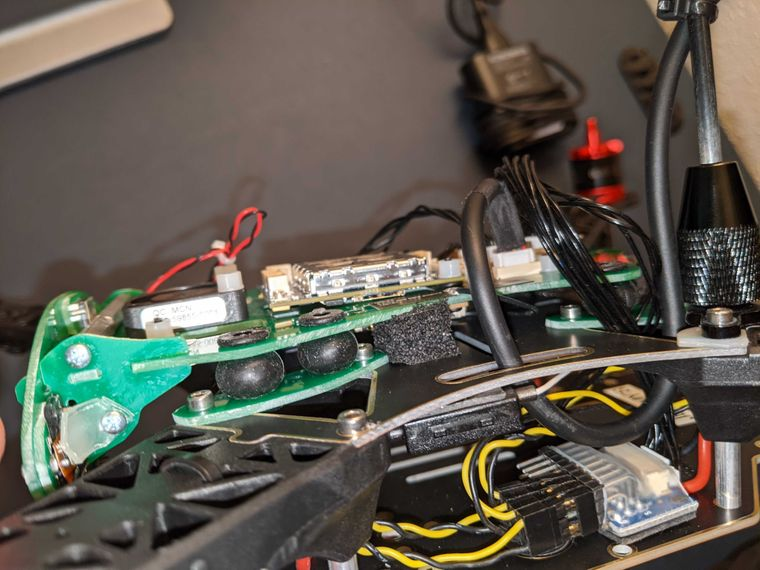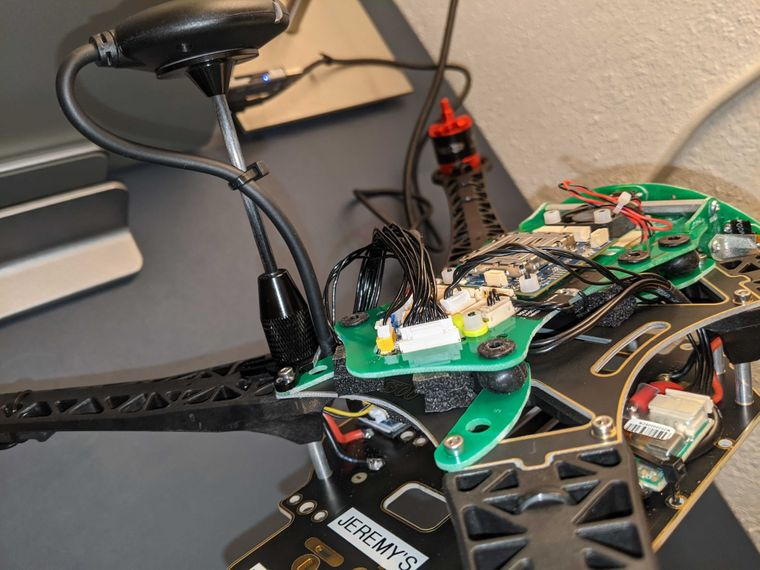• Thank for reply,
I'll try it in the tomorrow. We're in middle of night (GMT +7).

• if you have more advices, please tell us.
Best regard.

• Can you please send the serial number of the VOXL Flight PCB? We might be able to look up the calibration files in our production database?

• Hi @Chad-Sweet here is the serial number.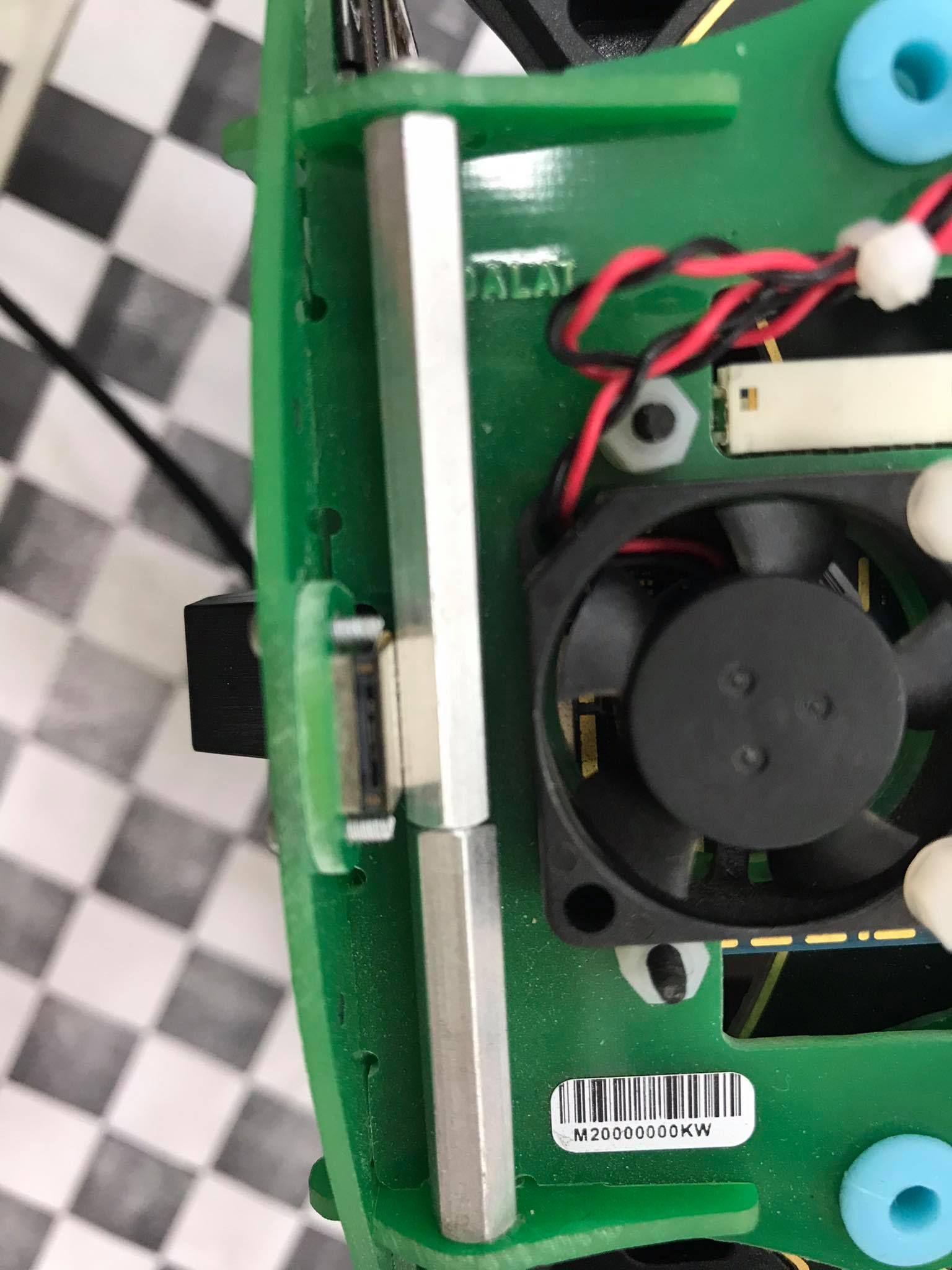• Here's the test data:

Stereo Camera Calibration

left_file:
image_width: 640 image_height: 480 camera_name: stereo/left camera_matrix: rows: 3 cols: 3 data: [492.469682, 0.000000, 287.720563, 0.000000, 492.799801, 241.856917, 0.000000, 0.000000, 1.000000] distortion_model: plumb_bob distortion_coefficients: rows: 1 cols: 5 data: [-0.171887, 0.054112, 0.000816, 0.000653, 0.000000] rectification_matrix: rows: 3 cols: 3 data: [0.999125, 0.024029, 0.034224, -0.023993, 0.999711, -0.001454, -0.034249, 0.000631, 0.999413] projection_matrix: rows: 3 cols: 4 data: [509.343373, 0.000000, 291.779423, 0.000000, 0.000000, 509.343373, 233.497673, 0.000000, 0.000000, 0.000000, 1.000000, 0.000000]

right_file:
image_width: 640 image_height: 480 camera_name: stereo/right camera_matrix: rows: 3 cols: 3 data: [494.055474, 0.000000, 337.960482, 0.000000, 494.170184, 223.591690, 0.000000, 0.000000, 1.000000] distortion_model: plumb_bob distortion_coefficients: rows: 1 cols: 5 data: [-0.182939, 0.076209, 0.000049, 0.002344, 0.000000] rectification_matrix: rows: 3 cols: 3 data: [0.999497, 0.010498, 0.029940, -0.010529, 0.999944, 0.000885, -0.029929, -0.001200, 0.999551] projection_matrix: rows: 3 cols: 4 data: [509.343373, 0.000000, 291.779423, -40.695510, 0.000000, 509.343373, 233.497673, 0.000000, 0.000000, 0.000000, 1.000000, 0.000000]

snav_file:
<SnavCalibration> <SnavCalibrationStereo> <SnavCalibrationLeftStereo> <param name="calib_pixel_width" value="640" /> <param name="calib_pixel_height" value="480" /> <param name="calib_memory_stride" value="640" /> <param name="principal_point_x" value="287.720563" /> <param name="principal_point_y" value="241.856917" /> <param name="focal_length_x" value="492.469682" /> <param name="focal_length_y" value="492.799801" /> <param name="distortion_0" value="-0.171887" /> <param name="distortion_1" value="0.054112" /> <param name="distortion_2" value="0.000816" /> <param name="distortion_3" value="0.000653" /> <param name="distortion_4" value="0.0" /> <param name="distortion_5" value="0.0" /> <param name="distortion_6" value="0.0" /> <param name="distortion_7" value="0.0" /> <param name="distortion_model" value="4" /> </SnavCalibrationLeftStereo> <SnavCalibrationRightStereo> <param name="calib_pixel_width" value="640" /> <param name="calib_pixel_height" value="480" /> <param name="calib_memory_stride" value="640" /> <param name="principal_point_x" value="337.960482" /> <param name="principal_point_y" value="223.59169" /> <param name="focal_length_x" value="494.055474" /> <param name="focal_length_y" value="494.170184" /> <param name="distortion_0" value="-0.182939" /> <param name="distortion_1" value="0.076209" /> <param name="distortion_2" value="0.000049" /> <param name="distortion_3" value="0.002344" /> <param name="distortion_4" value="0.0" /> <param name="distortion_5" value="0.0" /> <param name="distortion_6" value="0.0" /> <param name="distortion_7" value="0.0" /> <param name="distortion_model" value="4" /> </SnavCalibrationRightStereo> <param name="correction_factors_0" value="0" /> <param name="correction_factors_1" value="0" /> <param name="correction_factors_2" value="0" /> <param name="correction_factors_3" value="0" /> <param name="translation_0" value="-0.0798577979308862" /> <param name="translation_1" value="-0.000838769063517393" /> <param name="translation_2" value="-0.00239214571934757" /> <param name="rotation_0" value="0.00226741960521865" /> <param name="rotation_1" value="0.00433160780762917" /> <param name="rotation_2" value="-0.0134848065488883" /> </SnavCalibrationStereo> </SnavCalibration>

Tracking Camera Calibration

cal_file:
image_width: 640 image_height: 480 camera_name: tracking camera_matrix: rows: 3 cols: 3 data: [277.095893, 0.000000, 324.145728, 0.000000, 277.407080, 209.238122, 0.000000, 0.000000, 1.000000] distortion_model: fisheye distortion_coefficients: rows: 1 cols: 4 data: [-0.018687, 0.034754, -0.034056, 0.011701] rectification_matrix: rows: 3 cols: 3 data: [1.000000, 0.000000, 0.000000, 0.000000, 1.000000, 0.000000, 0.000000, 0.000000, 1.000000] projection_matrix: rows: 3 cols: 4 data: [303.434082, 0.000000, 333.786106, 0.000000, 0.000000, 297.322021, 188.396771, 0.000000, 0.000000, 0.000000, 1.000000, 0.000000]

snav_file:
<SnavCalibration> <SnavCalibrationDownward> <param name="calib_pixel_width" value="640" /> <param name="calib_pixel_height" value="480" /> <param name="calib_memory_stride" value="640" /> <param name="principal_point_x" value="324.145728" /> <param name="principal_point_y" value="209.238122" /> <param name="focal_length_x" value="277.095893" /> <param name="focal_length_y" value="277.40708" /> <param name="distortion_0" value="-0.018687" /> <param name="distortion_1" value="0.034754" /> <param name="distortion_2" value="-0.034056" /> <param name="distortion_3" value="0.011701" /> <param name="distortion_4" value="0" /> <param name="distortion_5" value="0" /> <param name="distortion_6" value="0" /> <param name="distortion_7" value="0" /> <param name="distortion_model" value="10" /> </SnavCalibrationDownward> </SnavCalibration>

• Thank for answer, I've checked the test data above. Here is the list of the /etc/snav# diectory and i checked the calibration.downward.xml and calibration.stereo.xml but both of them are similar as the test data from you.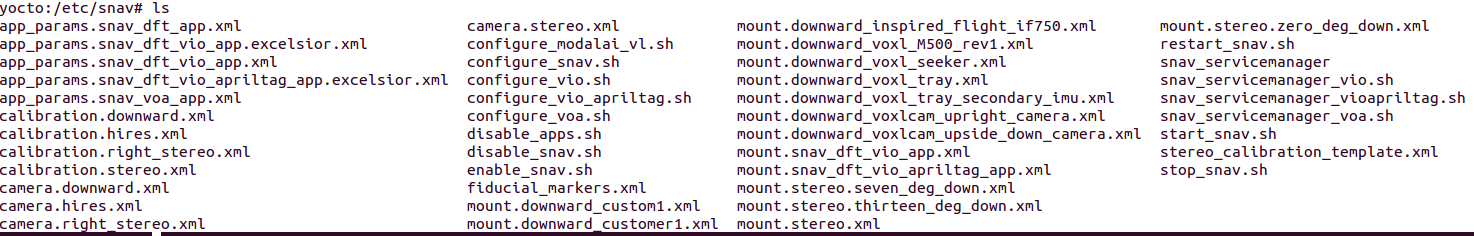• For the camera matrix i've checked in the ~/.ros/root/camera_info folder and they also non change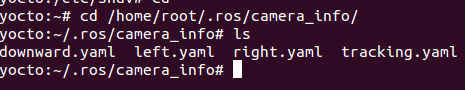• Ok, they should be exact. Did you remove vibration by adding foam?

• Yes, after adding some foam, The UAV now are stable than a bit. Do you think the battery should be problem? Our 3S battery are quite old.